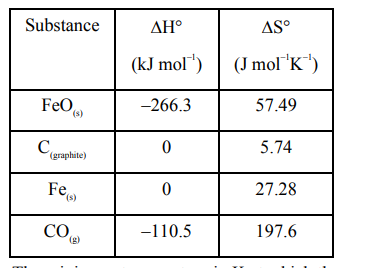# Data given for the following reaction is as follows:

Question:

Data given for the following reaction is as follows:

$\mathrm{FeO}_{(\mathrm{s})}+\mathrm{C}_{\text {(graplite) }} \longrightarrow \mathrm{Fe}_{(\mathrm{s})}+\mathrm{CO}_{(\mathrm{g})}$The minimum temperature in K at which the reaction becomes spontaneous is ________ .

Solution:

$\mathrm{T}_{\min }=\left(\frac{\Delta^{0} \mathrm{H}}{\Delta^{0} \mathrm{~S}}\right)$

$\Delta^{0} \mathrm{H}_{\mathrm{rxn}}=\left[\Delta_{\mathrm{f}}^{0} \mathrm{H}(\mathrm{Fe})+\Delta_{\mathrm{f}}^{0} \mathrm{H}(\mathrm{CO})\right]-$

$=\left[\Delta_{\mathrm{f}}^{0} \mathrm{H}(\mathrm{Fe} \mathrm{O})+\Delta_{\mathrm{f}}^{0} \mathrm{H}\left(\mathrm{C}_{\text {(graphite) }}\right)\right]$

$=[0-110.5]-[-266.3+0]$

$=155.8 \mathrm{~kJ} / \mathrm{mol}$

$\Delta^{0} \mathrm{~S}_{\mathrm{rxn}}=\left[\Delta^{0} \mathrm{~S}(\mathrm{Fe})+\Delta^{0} \mathrm{~S}(\mathrm{CO})\right]-$

$\left[\Delta^{0} \mathrm{~S}(\mathrm{Fe} \mathrm{O})+\Delta^{0} \mathrm{~S}\left(\mathrm{C}_{\text {(graphite) }}\right)\right]$

$=[27.28+197.6]-[57.49+5.74]$

$=161.65 \mathrm{~J} / \mathrm{mol}-\mathrm{K}$

$\mathrm{T}_{\min }=\frac{155.8 \times 10^{3} \mathrm{~J} / \mathrm{mol}}{161.65 \mathrm{~J} / \mathrm{mol}-\mathrm{K}}=963.8 \mathrm{~K}$

$\simeq 964 \mathrm{k}$ (nearest integer)